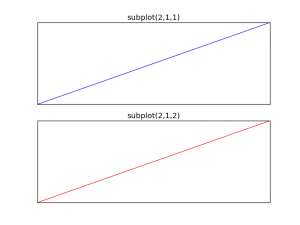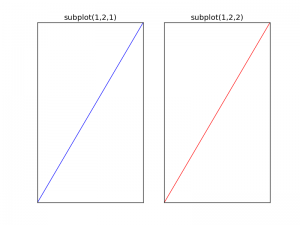# Matplotlib Subplot

Python hosting: Host, run, and code Python in the cloud!

The Matplotlib subplot() function can be called to plot two or more plots in one figure. Matplotlib supports all kind of subplots including 2x1 vertical, 2x1 horizontal or a 2x2 grid.

Related courses

• Data Visualization with Matplotlib and Python

• Horizontal subplot
Use the code below to create a horizontal subplotmatplotlib subplot

Vertical subplot
By changing the subplot parameters we can create a vertical plotmatplotlib subplot vertical

Subplot grid
To create a 2x2 grid of plots, you can use this code: